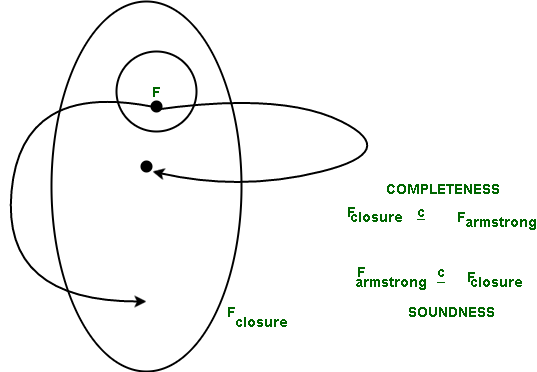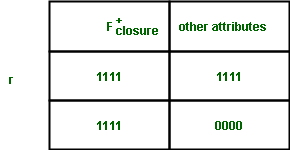Related Articles
Proving Correctness of Armstrong’s Axioms
• Difficulty Level : Expert
• Last Updated : 21 Jun, 2020

Prerequisite – Armstrong’s Axioms in Functional Dependency in DBMS
Armstrong mentioned that rules 1 through 3 have completeness along with soundness. Armstrong’s axioms are complete because for a given set of functional dependencies, F, all functional dependencies implied by F+ can be derived from F using these rules.

What we need to prove –
The set of functional dependencies that can be derived with logic from a given set of functional dependencies(Fclosure) and the set of functional dependencies that can be inferred from Armstrong’s Axioms (Farmstrong) are same. In terms of sets, if we want to show equality, then we write it as, one is a proper subset of the other. Hence, we need to show that,

Fclosure  is a proper subset of Farmstrong

Correctness means that whatever can be derived from F can also be derived using Armstrong’s Axioms.Fclosure+ represents the closure of F. Mathematically we can represent, Fclosure+ = {A| Fclosure+ implies A can be derived from F using Armstrong’s Axioms} where, A belongs to Real Numbers.

Claim-1 :is derivable from F using Armstrong’s Axioms if and only if Y is a proper subset of Fclosure+.

To prove ‘if’ part :
Let Y={A0, A1, ……, Am, } where Y is a proper subset of Fclosure+. Fclosure+ implies Aj can be derived from F using Armstrong’s Axioms} where, A belongs to 0<j<=m
If we follow union rule then,is derivable from F

To prove the ‘only if’ part :is derivable from F using Armstrong's Axioms
by projection rule we know that Fclosure+ implies Ajwhere, A belongs to 0<j<=m
Thus by the property of Fclosure+, Aj belongs to Fclosure+.
This implies that Y is a proper subset of Fclosure+.

X determines Y follows from F using Armstrong’s Axioms.
We shall try to prove the contrapositive of the above statement.
We would prove that X determines Y cannot be determined from F using Armstrong’s Axioms. In terms of tuple Relations we can say that, There exists an instance r belonging to Real numbers such that, all the functional dependencies of F hold on r. However, X implies Y does not hold.
This mentions that our assumption was wrong and the claim stands correct.
Hence proved that Claim 01 is correct.

Let us consider that r has only 2 rows and is represented by r{Fclosure+ attributes, other attributes}Claim-2 :
All FDs of F are satisfied by r. Let us again take the negative of it. Let W implies R belonging to F does not satisfy r, then W is a proper subset of Fclosure+ and Z is not a proper subset of Fclosure+.
Let G belongs to Z- Fclosure+

We get :because W is a proper subset of Fclosure+,by rule of transitivity,by rule of reflexivity as G belongs to Z andby rule of transitivity.

By definition of closures G must belong to Fclosure+
We had taken a contradiction which was proven wrong, which means that the statement is true.
Hence proved that Claim 02 is correct.

Claim-3 :
X implies Y is not satisfied by r.
We assume the contradiction again (X does not imply Y). Due to the Structure of r, Y is a proper subset of Fclosure+ which means that X implies Y can be proved using Armstrong’s Axioms.
Our assumption is proved false hence the claim is correct.
Hence proved that Claim 03 is correct.

These mean that when X does not imply Y, using Armsrong’s Axioms, then F doesn’t logically imply that X implies Y.
We can also say that when X implies Y, using Armsrong’s Axioms (mechanically), then F logically implies that X implies Y as well.
Hence proved that Armstrong’s Axioms are complete.

Attention reader! Don’t stop learning now. Get hold of all the important CS Theory concepts for SDE interviews with the CS Theory Course at a student-friendly price and become industry ready.

My Personal Notes arrow_drop_up
Recommended Articles
Page :